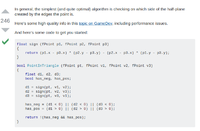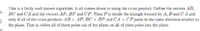# how to check which side of a line a point lies on

#### ignoremail

##### New member
There's plenty of answers for this online but I just don't seem to understand them. How, given a line say AB where you know points A and B, can you find whether a point P lies above or below the line. I've seen people using formulas involving dot products and such but I'm unsure why they are using them.

#### Subhotosh Khan

##### Super Moderator
Staff member
There's plenty of answers for this online but I just don't seem to understand them. How, given a line say AB where you know points A and B, can you find whether a point P lies above or below the line. I've seen people using formulas involving dot products and such but I'm unsure why they are using them.
That will depend on the definition of "above" and "below" of the "line".

How would you define above or below for a "vertical" line?

How would you define above or below for a "slant" line?

•Jomo

#### Dr.Peterson

##### Elite Member
There's plenty of answers for this online but I just don't seem to understand them. How, given a line say AB where you know points A and B, can you find whether a point P lies above or below the line. I've seen people using formulas involving dot products and such but I'm unsure why they are using them.
There are several ways we could try to help you; but since you are specifically confused about what you have seen, it will be helpful if you can quote at least one of those, and what you are unsure of. Then we can directly answer your question about "why they are using them", based on knowledge of what they are actually doing.

On the other hand, it may be that in your context, vectors are not the appropriate way to answer such a question in the first place. So another thing that would be helpful is your context: what are the specifics of the problem(s) you are trying to solve yourself (e.g. what data are you given, and what is the ultimate goal), as well as what you are learning and/or working on?

#### ignoremail

##### New memberThis was one of the examples I seen and I'm not sure what the first equation at the top represents and works out. I understand that it is calculating the sign, but how is it doing that. Another example I seen wasI probably should have mentioned its more specific and about finding if a point lies in a triangle, but I broke it down into finding which side a point lies on, which I understand you just repeat for each vertex and compare them. Its the equations to do this that I don't really understand.

#### ignoremail

##### New member
That will depend on the definition of "above" and "below" of the "line".

How would you define above or below for a "vertical" line?

How would you define above or below for a "slant" line?
for example, given a triangle ABC, above AB is outside the triangle, Above BC is outside the triangle etc

#### Dr.Peterson

##### Elite Member
for example, given a triangle ABC, above AB is outside the triangle, Above BC is outside the triangle etc
That doesn't really make sense. What if AB is the bottom of the triangle??? It appears that you are not really asking about "above" or "below" at all. That could be part of your problem, or you may just not have clearly stated what you are looking for.

In terms of a triangle, we have to define "inside"; one convenient way is to say that P is inside ABC when it is on the same side of AB as C, on the same side of BC as A, and on the same side of CA as B. This will be the key to understanding the algorithm.

This was one of the examples I seen and I'm not sure what the first equation at the top represents and works out. I understand that it is calculating the sign, but how is it doing that.
For context, I see that this is in https://stackoverflow.com/questions/2049582/how-to-determine-if-a-point-is-in-a-2d-triangle, and is in response to a question about determining whether a point lies in a triangle. I also see that issues have been raised about what happens when a point outside the triangle lies on one of the (extended) edges. But I'll just comment on the main idea.

The function "sign" returns twice the signed area of the triangle P1P2P3 (that is, positive in one orientation, negative in the other); for one source, see the last formula under Modern Triangles here. That can be taken as determining whether P3 is on the left or the right of P1P2.

Given that, and my definition above, can you see what is being done?